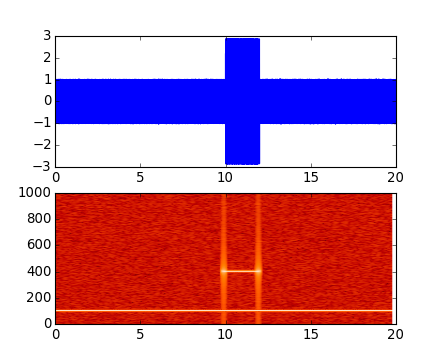#### Previous topic

pylab_examples example code: simple_plot_fps.py

#### Next topic

pylab_examples example code: spectrum_demo.py

# pylab_examples example code: specgram_demo.py¶```#!/usr/bin/env python
from pylab import *

dt = 0.0005
t = arange(0.0, 20.0, dt)
s1 = sin(2*pi*100*t)
s2 = 2*sin(2*pi*400*t)

# create a transient "chirp"
mask = where(logical_and(t>10, t<12), 1.0, 0.0)

# add some noise into the mix
nse = 0.01*randn(len(t))

x = s1 + s2 + nse # the signal
NFFT = 1024       # the length of the windowing segments
Fs = int(1.0/dt)  # the sampling frequency

# Pxx is the segments x freqs array of instantaneous power, freqs is
# the frequency vector, bins are the centers of the time bins in which
# the power is computed, and im is the matplotlib.image.AxesImage
# instance

ax1 = subplot(211)
plot(t, x)
subplot(212, sharex=ax1)
Pxx, freqs, bins, im = specgram(x, NFFT=NFFT, Fs=Fs, noverlap=900,
cmap=cm.gist_heat)
show()
```

Keywords: python, matplotlib, pylab, example, codex (see Search examples)